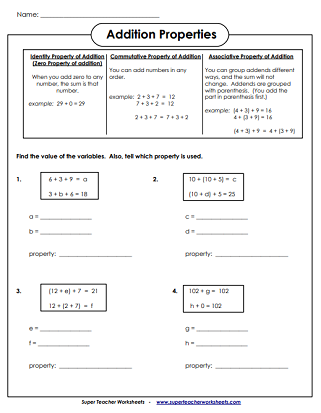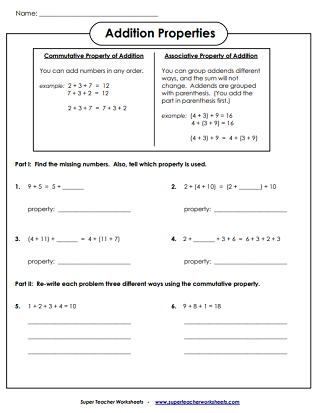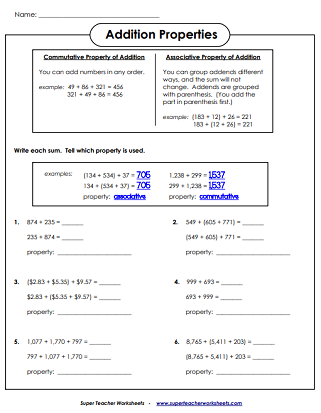## Basic

Practice recognizing and working with the properties of addition problems. This is a good introductory worksheet that contains only simple 1 and 2-digit numbers.
This worksheet allows students to practice working with the properties of addition using only basic numbers.
This worksheet includes the associative, commutative, and the identity (zero) properties. Students must write the value of each variable.

## Intermediate

This intermediate-level worksheet features larger numbers into the thousands and money amounts.

Practice adding with 2, 3, and 4-digit numbers.

Add several 2, 3, and 4-digit numbers together by lining them up into columns.

Math Worksheets

S.T.W. has thousands of worksheets. Visit the full math index to find them all, sorted by topic.

## Worksheet ImagesMy Account
Site Information